# Books and Worked Examples

### Kindle Editions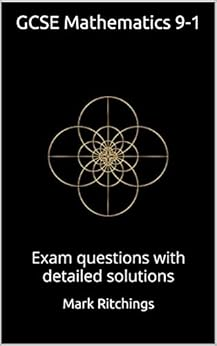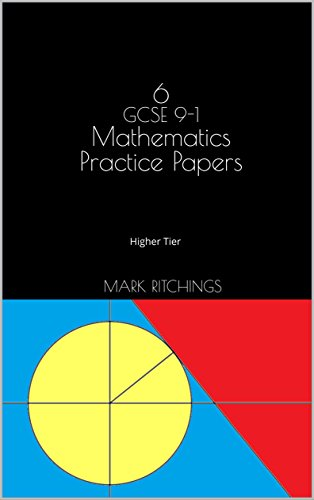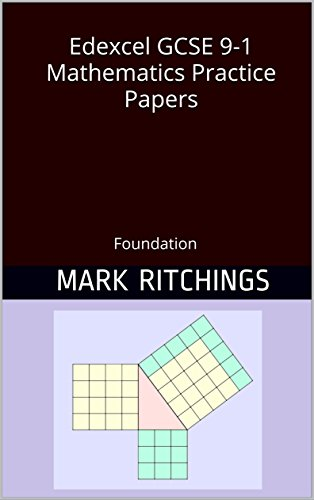### Paperbacks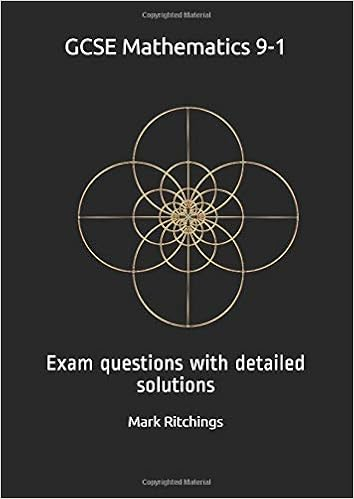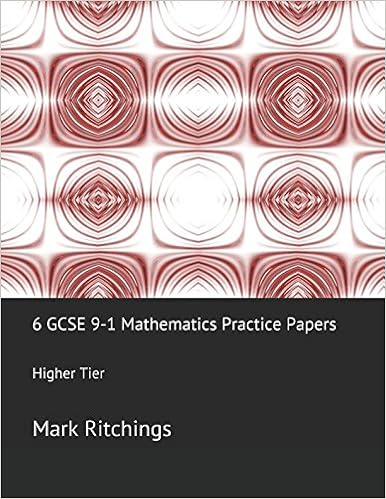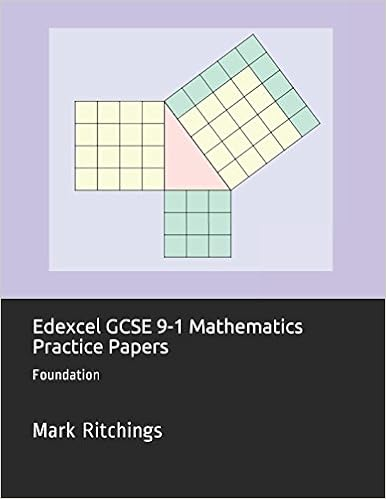### KS3

A year 8 homework question

### GCSE

Edexcel June 13 C q6
Edexcel Nov 13 NC q21
Edexcel Nov 14 C q16
Edexcel Sample assessment material q16
Edexcel Sample assessment material q24
Edexcel Sample question on functions
Edexcel Sample question on sine rule cosine rule and area
GCSE question - circle, tangent, area
GCSE maths question - cosine rule
An area problem (sector, segment, triangle, circle)
A geometry proof
Similar shapes, area
Edexcel sample question (Trigonometry, Pythagoras, area)
Standard Form Questions
Probability
Geometric Ratio Vector Problems

### A level

An O level question from 1976
A challenging question?
An area problem
Volume of revolution
An A level question plus a bit about Lambert's function
Induction question

### Statistics

AQA S1 Normal distribution
AQA S2 Continuous Random Variable

### C3

C3 Edexcel June 2016 q 9
C3 Composite functions
C3 trigonometry

### C4

C4 Binomial expansion 2013 (R)
C4 Differential Equations June 2005
C4 Parametric Equations
C4 Parametric Equations Edexcel Review 2 question 76
C4 January 2012 6c integration by substitution
C4 January 2008 8 differential equation
C4 June 2017 8 parametric equations and integration
C4 June 2015 7 partial fractions differential equations
C4 June 2014 7 Parametric Integration

An application of differentiation question

### Edexcel Core Pure 2

Roots of polynomials

### AQA FP1

Series - Method of differences

### Edexcel Statistics

Poisson distribution - hypothesis test

2006 HSC

### STEP

An old STEP question
and its solution

### Calculus of Variations

Example 1
Example 2
M820 Exercise 5.23

Example 1
Example 2
Example 3

### Nonlinear Ordinary Differential Equations

M821 Jordan and Smith, Nonlinear Ordinary Differential Equations Exercise 1.2
M821 J&S Exercise 1.5
M821 J&S Exercise 1.6
M821 J&S Exercise 1.7